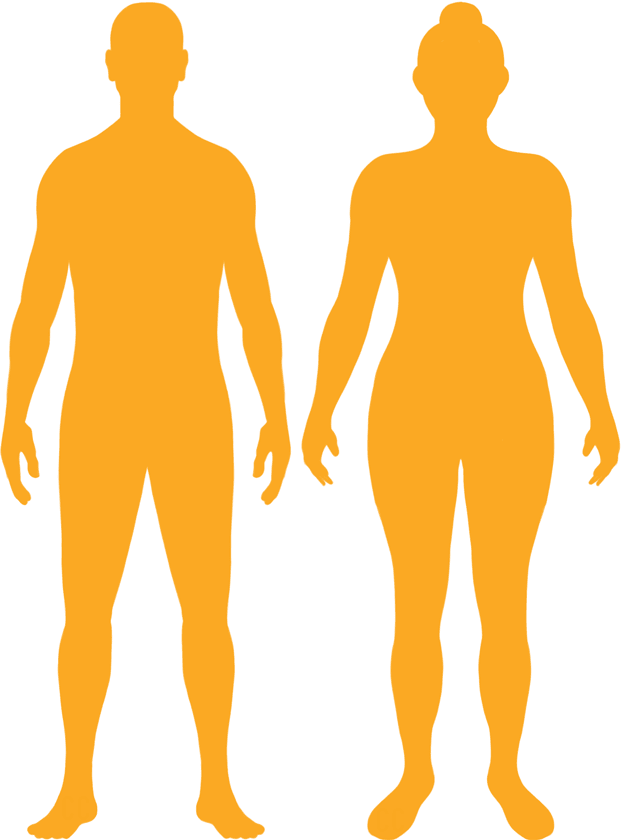# BMI Calculator

Result for 7'9" and 326 lbs.
Change Here.26.5

### Overweight BMI

At 7'9" and 326 Pounds your BMI is 26.5

### BMI Scale

 Under Normal Over Obese
-19 lbs
to reach a
normal BMI classification.
##### Normal BMI Ends:
307.5 lbs
+43 lbs
until you reach an obese BMI classification.
369.1 lbs

### BMI Grading Table2 for Height 7'9"

 Weight Weight Class Below 227.6 lbs Underweight 227.6 lbs - 307.5 lbs Normal Weight 307.5 lbs - 369.1 lbs Overweight 369.1 lbs and Above Obese

### Weight Loss

If you were seeking a normal BMI weight of 307.5lbs, and you lost two pounds a week4, then you could reach a normal BMI in
9.5 Weeks

### Overweight BMI Silhouette### Ideal Weight for a 7 foot 9 Male or Female2

The ideal weight range using the BMI for a male or female with a height of 7'9" is

### Between

227.6 lbs

and
307.5 lbs
How to Calculate BMI3 for 7'9" and 326 lbs
(7 x 12) + 9 = 93
(326 / (93 x 93)) x 703
= 26.49762978379
7 = foot | 9 = inch | 326 = weight lbs

### Conversion

• 7 foot 9 = 93 inches
• 7 foot 9 = 236.22cm
• 326 pounds = 23 stone 4 pounds
• 326 pounds = 147.87 kg
• 326 pounds = 147871.11 grams

### 7'9" and 326 lbs Summary

What is the BMI for a 7'9" and 326 lbs female? 26.5 BMI, Overweight.
What is the BMI for a 7'9" and 326 lbs male? 26.5 BMI, Overweight.
What is the ideal weight for a 7'9" female? Between: 227.6lbs and 307.5lbs
What is the ideal weight for a 7'9" male? Between: 227.6lbs and 307.5lbs
If I am 7ft 9in and weigh 326 lbs, is that a good weight for my height? Under the BMI classification, 326 lbs is classed as being Overweight.